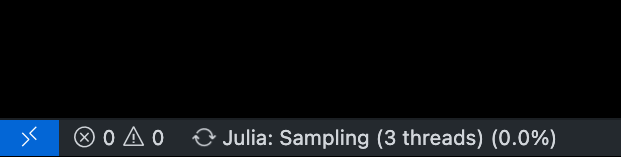# Sampling percentage indicator

Why this don’t show percentage with interval of 1. I mean like 1%, 2%… but it shows directly around 50%. Is there any way to change this ? I feel like sitting blind, when running long inferences.Which package/function are you using? Can you post a minimal example of code that result in that behaviour?

You can run this example first with MCMCSerial and then MCMCThreads. It will show you what I want. In case of serial you will see percentage updating continuously while in case of MCMCThreads it happens directly in end. For short programs thats ok but for long running programs its always good to have some estimate like how long it gonna take or how much is done.

“I’m running program as Julia text file in vs-code via REPL and not in jupyter notebook”

``````# Import libraries.
using Turing, StatsPlots, Random

# Set the true probability of heads in a coin.
p_true = 0.5

# Iterate from having seen 0 observations to 100 observations.
Ns = 0:100

# Draw data from a Bernoulli distribution, i.e. draw heads or tails.
Random.seed!(12)
data = rand(Bernoulli(p_true), last(Ns))

# Declare our Turing model.
@model function coinflip(y)
# Our prior belief about the probability of heads in a coin.
p ~ Beta(1, 1)

# The number of observations.
N = length(y)
for n in 1:N
# Heads or tails of a coin are drawn from a Bernoulli distribution.
y[n] ~ Bernoulli(p)
end
end

# Settings of the NUTS() sampler.
iterations = 1000000

# Start sampling.
chain = sample(coinflip(data), NUTS(), MCMCSerial(),iterations, 2)

chain = sample(coinflip(data), NUTS(), MCMCThreads(),iterations, 2)
``````
1 Like